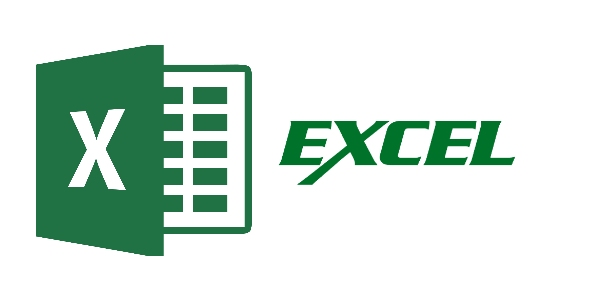# Post Test Excel 2007 And 2010 Intermediate

13 Questions | Attempts: 175
ShareSettings• 1.
Default extension files for Excel 2007/2010 is
• A.

Docx

• B.

Pptx

• C.

Exls

• D.

Xlsx

• 2.
Shortcut key for Print Preview in Excel 2010 is
• A.

Ctrl + F2

• B.

Alt + F2

• C.

Shift + F2

• D.

Ctrl + Alt + F2

• 3.
One of features that help user make using spreadsheet with quicker and faster is Insert Function Utility. Where i sthe location for Insert Function Utility key?
• A.

Formula Tab --> within Function Library group

• B.

Formula Tab --> within Defined Named group

• C.

Insert Tab --> within Function Library Group

• D.

Insert Tab --> within Defined Named group

• 4.
Formula to create current date and time is
• A.

=DATE(NOW)

• B.

=DAY(NOW)

• C.

=NOW()

• D.

=(DATE)

• 5.
If the number is 364.66667, what is the final number if we use formula =ROUND(cell that contain 364.66667,0)?
• A.

365

• B.

364.7

• C.

364

• D.

364.6

• 6.
What formula to combine several text at several cell into one cell?
• A.

=CONSOLIDATE()

• B.

=CONCATENATE()

• C.

=TRIM()

• D.

=MID()

• 7.
WHAT IS THE LAST COLUMN AND ROW FOR EXCEL 2007/2010?
• A.

IV, 65536

• B.

XFD, 1048576

• C.

Unlimited

• D.

ZZZ, 999999

• 8.
Which formula is false in Excel 2007/2010?
• A.

=SUMIF(D4:D10,">600")

• B.

=ROUNDUP(C2/C3,1)

• C.

=IF(AVERAGE(B3:B6)>25,SUM(B3:B6),0)

• D.

=LEFTS(D4)

• 9.
What symbol represent absolute reference in Ms Excel?
• A.

\$

• B.

&

• C.

%

• D.

#

• 10.
There are pivot graph and pivot table to present large data in real time in excel 2007/2010.
• A.

True

• B.

False

• 11.
What result for the following formula? =if(c2>500,"good","not good") where value in c2=500
• A.

Not good

• B.

Good

• C.

Not valid

• D.

No result appear

• 12.
Formula's that can find any value related with finance are
• A.

SUM(), LEFT(), DSUM()

• B.

FV(), CONCATENATE(), NOW()

• C.

FV(), NPV(), PV()

• D.

DAY(), Now(), Today()

• 13.
What exactly year 2001! means in excel 2007/2010?
• A.

Name of cell reference

• B.

Name of worksheet

• C.

Absolute reference

• D.

One of function in math and trig

## Related TopicsBack to top
×

Wait!
Here's an interesting quiz for you.